International Journal of Energy and Power Engineering
Volume 5, Issue 3, June 2016, Pages: 97-104

Modelling and Analysis of Thermoelectric Generation of Materials Using Matlab/Simulink

K. P. V. B. Kobbekaduwa, N. D. Subasinghe*

National Institute of Fundamental Studies, Hanthana Road, Kandy, Sri Lanka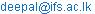(N. D. Subasinghe)

*Corresponding author

K. P. V. B. Kobbekaduwa, N. D. Subasinghe. Modelling and Analysis of Thermoelectric Generation of Materials Using Matlab/Simulink. International Journal of Energy and Power Engineering. Vol. 5, No. 3, 2016, pp. 97-104. doi: 10.11648/j.ijepe.20160503.12

Received: May 2, 2016; Accepted: May 18, 2016; Published: May 19, 2016

Abstract: This paper presents several models and implementations on measuring the thermoelectric behaviour of an unknown material using Matlab/Simulink. The proposed models are designed using Simulink block libraries and can be linked to data obtained from an actual experimental setup. This model is unique, as it also contains an implementation that can be used as a laboratory experiment to estimate the thermal conductivity of the unknown material thus, making it easy to use for simulation, analysis and efficiency optimization of novel thermoelectric material. The model was tested on a natural graphite sample with a maximum output voltage of 0.74mV at a temperature difference of 25.3K. Thus, according to the collected data, an experimental mean value of 68W/m.K was observed for the thermal conductivity while the Seebeck coefficient had a mean value of -3.1µV/K. Hence, it is apparent that this model would be ideal for thermoelectric experimentation in a laboratory based environment especially as a user interface for students.

Keywords: Seebeck Effect, Thermoelectric Power, Thermal Conductivity, Electrical Conductivity, Simulink Modelling

Contents

1. Introduction

Thermoelectric effect is a simple phenomenon based on the thermal and electrical characteristics of a material. Discovered in the early 1800s it was thought to be an interesting form of energy conversion that relies on the physical characteristics of materials. This phenomenon is observed when two different types of material are combined and a temperature gradient is applied between the joint and open ends. When the fore mentioned conditions are met, a thermal current flows through the combined thermocouple, hence a voltage is generated between them. The thermoelectric effect, generally known as the Seebeck effect, gives rise to this inherent EMF or voltage due to the material property known as the Seebeck coefficient. These individual thermocouples can be combined in series to increase the output voltage to create a single thermoelectric module commonly known as a Peltier module. These can be used as voltage generators known as Thermoelectric Generators (TEG) or as coolers known as Thermoelectric Coolers (TEC). The main advantage of thermoelectric power is, it is a solid state energy conversion that does not have mechanical or liquid based moving parts. Hence, modules can be designed to be compact, stable as well as being reliable and noiseless. The main drawback is the efficiency of these materials thus, their uses have been confined to relatively smaller applications. However, when it comes to energy scavenging, thermoelectric generators always improve the overall energy efficiency of an existing system. For example, certain car manufactures could increase the fuel efficiency by over 5% simply generating electricity from the exhaust heat . With the advent of semiconductor materials as well as improvements in synthesis, materials with larger efficiency values have been discovered in the recent past, hence the renewed interest in this form of energy conversion. This has resulted in a plethora of new applications in numerous fields such as the automotive industry, space exploration and wearable nano-based technology.

Modelling of TE device or the behaviour of individual materials is an important prerequisite for the design and control verification of the final output device. Hence, the model has to be integrated seamlessly in to the overall system model that may contain other electrical, thermodynamic, or even mechanical components . Numerous research work on modelling a thermoelectric material or module has been done using software such as SPICE [3, 4] as well as Dynamic and static modelling of TE modules using MATLAB/Simulink [5, 6]. The modelling of thermal and power generation behaviour of these TEGs have been extensively studied  and the output values have also been modelled and estimated using novel techniques such as Artificial Neural Networking . In most of these prior work the main focus has been on modelling TEG modules where as in this research we focus on modelling the behaviour of a particular material.

2. Principle of Thermoelectric Generation

The physical process of the Seebeck effect can be characterized in 5 distinct steps,

Temperature difference generates a difference in Fermi level

Bandgap distance changes with temperature

Diffusion coefficient is a function of temperature

Charge carriers move from the heated side to cold side - thermodiffusion

Electric field will be generated due to the transport of charge carriers

In this paper we specifically look at modelling the TEG of materials. The following equations govern the thermoelectric behaviour of any material [9,10]. The Seebeck coefficient S is defined as: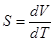(1)

where V is the Seebeck voltage or electromotive force (EMF) and T is the temperature. Apart from the Seebeck effect there are 3 other forms of energy conversion taking place in a TEG material these are, thermal conduction described by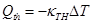(2)

where κTH is the thermal conductivity of the material and ΔT = TH (hot side temperature) −TC (cold side temperature). Joule heating, which is the heat dissipation due to the internal resistance of the material given by,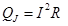(3)

where R is the electrical resistance and I is the current. Peltier cooling/heating effect, which is a phenomenon of heat absorption/dissipation by a junction between two dissimilar materials when electrical current flows through the junction is given by,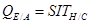(4)

Apart from the above the additional Thompson effect, which is described by the Thompson coefficient Ʈ= dS/dT is small enough to be neglected. Thus, heat flow at the hot and cold end respectively can be expressed as,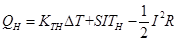(5)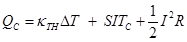(6)

Thus, the net power is given by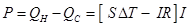. Hence, the output voltage is,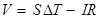(7)

Apart from the output values the usefulness of a thermoelectric material is dependent on the power factor of the said material this is calculated using the Seebeck coefficient and the electrical conductivity. Thus power factor Pf is given by,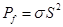(8)

The efficiency of a thermoelectric material is described using a dimension less figure of merit Z. A good TEM must combine a large Seebeck coefficient S with high electrical conductivity σ and low thermal conductivity κTH. Hence FOM is given by,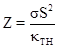(9)

The efficiency of these materials is described according to the output electric power compared to the applied heat energy QH. Thus,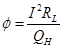(10)

where RL is the load resistance. Using the FOM value the maximum efficiency of the TE device is written as,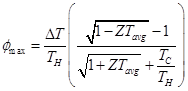(11)

where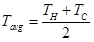3. Measurement of Thermal Conductivity

There are several methods to measure thermal conductivity. The measurement of heat flow is done directly which is known as absolute method and indirectly known as comparative method. Apart from measuring heat flow there are several other methods to measure thermal conductivity at sub ambient temperatures and higher. The most commonly used method is the axial flow type where heat flow is considered as axial and conductivity calculated accordingly.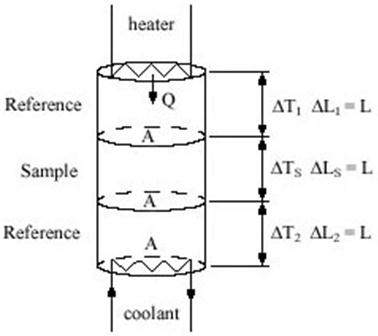Figure 1. Comparative cut bar method for measuring thermal conductivity .

In this paper we consider the comparative cut bar method which is widely used for determining axial thermal conductivity.

In this, the principle of the measurement lies with passing the heat flux through a known sample and an unknown sample and comparing the respective thermal gradients, which will be inversely proportional to their thermal conductivities [11, 12]. As shown in figure 1 this method involves the measurement of 4 separate temperature values T1, T2, T3, T4

where,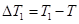,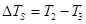and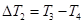. Thus, the heat flux can be calculated as,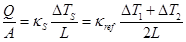(12)

where κS and κref are thermal conductivities of the sample and reference material respectively. Thus, κS can be calculated as,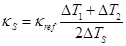(13)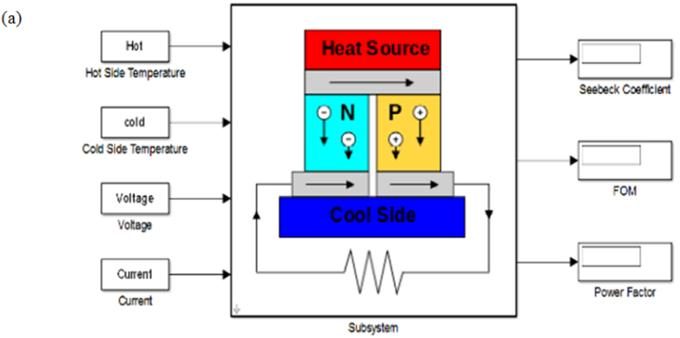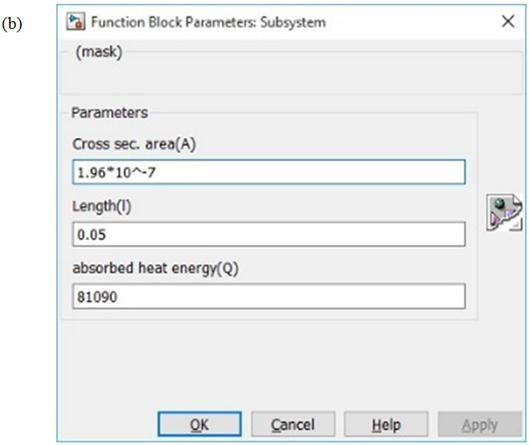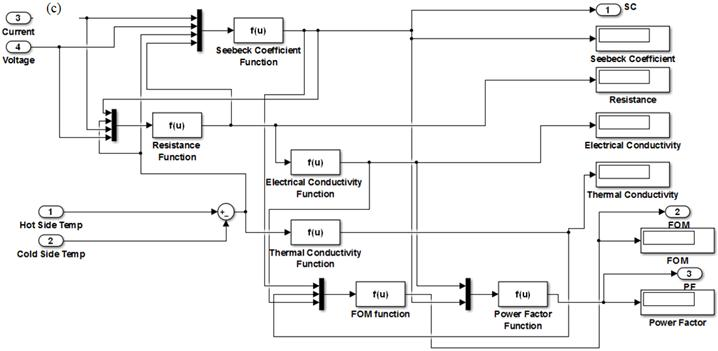Figure 2. (a) Mask implementation, (b) Initial conditions and (c) Subsystem of the proposed TE model.

4. Model Building and Implementation

The required data about the material should be obtained experimentally and the model is a guideline to implement and find the pertinent values related to thermoelectricity i.e. Seebeck coefficient, Power Factor and the figure of merit. MATLAB/Simulink software is used to construct the model. Previous work  includes a model based on the specifications related to Peltier modules, hence this paper looks at the feasibility of using an extended model to calculate the important factors related to thermoelectric behaviours of a novel material. A secondary model is used for calculating the thermal conductivity of the material based on the comparative cut bar method mentioned previously.

Figure 2 shows that in the initial theoretical model resistance is also calculated from the current, voltage and temperature data as well as the user inputs. In this case the thermal conductivity value also needs to be calculated using a given absorbed heat energy value. As the accuracy of this value is questionable (due to various heat loss factors) it is better to use a tested method as mentioned above to calculate the thermal conductivity. Thus, in this model we have used the aforementioned comparative cut bar method to find an experimental value for thermal conductivity. The standalone Simulink implementation for this method is as shown in figure 3 below.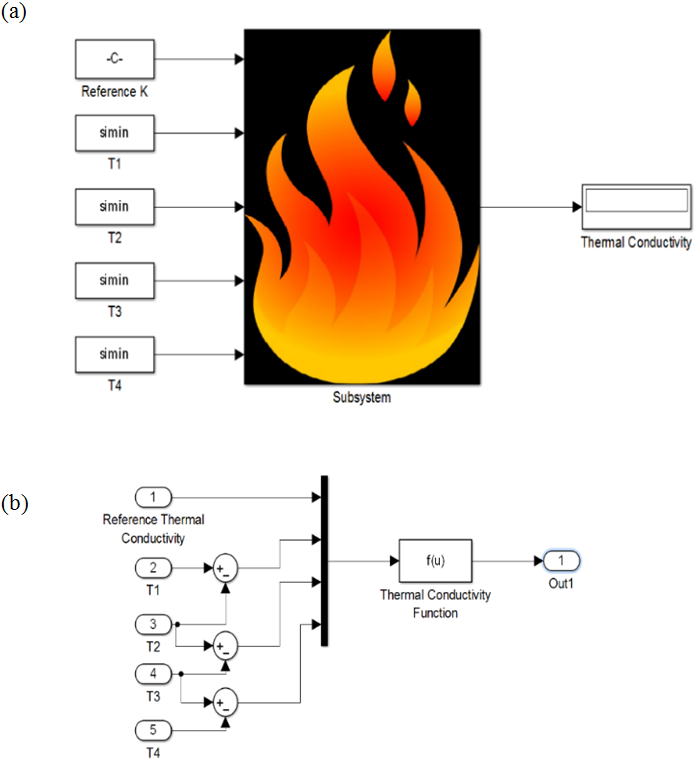Figure 3. (a) Masked implementation and (b) Subsystem of thermal conductivity model.

In this model we need 4 separate temperature measurements along with the thermal conductivity of the reference material used in the experiment. Thus, the front view and the parameter block used to enter the above values is shown in figure 4 (a) and (b) respectively. We can combine both these models to obtain the final implementation and the complete subsystem as seen in figure 5. Thus, the reference material thermal conductivity, length and cross sectional area of the sample are given as initial conditions and entered in the dialog box. In this revised model resistance can be added as a set of measured data instead of a theoretical calculation. The thermal conductivity implementation is added to the subsystem of the final model. The data is read off the MATLAB workspace and by adjusting the ‘simulation stop time’ we can observe the resulting values at each of the measured data points. To obtain the Seebeck coefficient, FOM and Power factor of a given data set the inputs should be obtained from the MATLAB workspace hence we can observe variations in the final output values. The fore mentioned outputs are obtained according to the equations 1 through 13.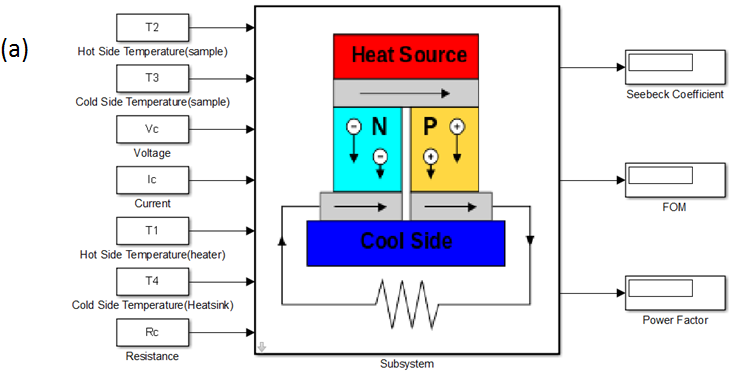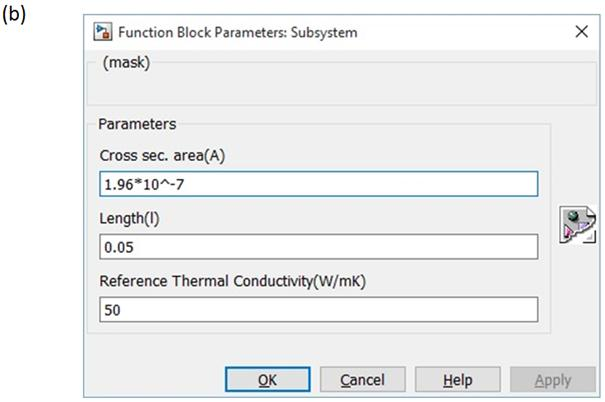Figure 4. The (a) masked implementation, (b) Parameter block for the thermoelectric model.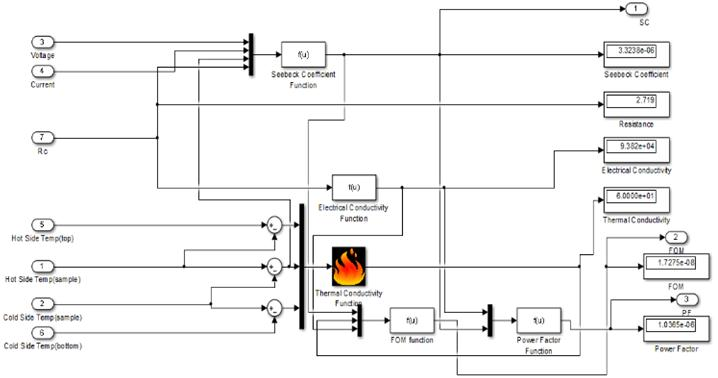Figure 5. The subsystem for the thermoelectric model combined with the model for thermal conductivity.

5. Simulation

The model is used to simulate and find the Seebeck coefficient of a graphite sample which has dimensions of 4mm thickness and 1.17cm diameter (A = 1.075cm2). This sample is created using a powdered graphite sample ground using a ball mill and then compressed at 1.5 ton/m2 pressure. The reference samples are soldering lead melted and solidified to form a sample which has similar dimensions as above. The setup is insulated to reduce thermal leakage using wood and cotton. The experimental setup is as shown below in figure 6.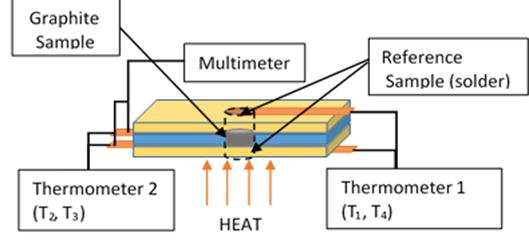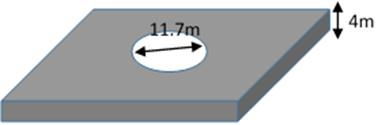Figure 6. Experimental setup to measure temperature values and output voltage and current.

The thermal conductivity value of solder lead (Sn 63% Pb 37%) used (κreff) is 50 W/mK . Using the above set up voltage, current and resistance values are obtained along with 4 temperature values. Figure 7 shows variations of the fore mentioned values with varying temperature difference for the graphite sample while the VI curve is shown in figure 8.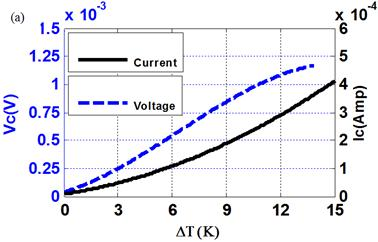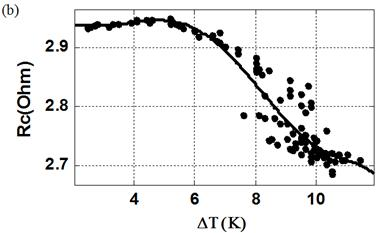Figure 7. (a) Output voltage, Current (b) Resistance vs. Temperature difference.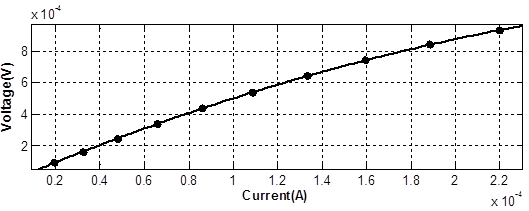Figure 8. VI curve related to the graphite sample.

In this example we have measured and collected a data set which has 100 points. By reading the obtained values from the MATLAB workspace we can estimate values for thermoelectric generation of the sample. Figure 9 (a) and (b) shows the thermal conductivity and Seebeck coefficient values obtained at several different temperature differences for the natural graphite sample

From the graphs in figure 9 we can observe that the thermal conductivity varies with temperature difference with a maximum value of 95W/m.K and a mean value of 68W/m.K. These values are in line with previously observed thermal conductivity values of graphite ranging from 25-470W/m.K [14, 15].

The Seebeck coefficient varies between 0 and -10µV/K with a mean value of -3.1µV/K. Values for the Seebeck coefficient of graphite have been previously reported for flexible graphite , Carbon nanotubes  and Graphite Intercalation compounds and composites [18, 19] and in most cases they vary from positive to negative due to the presence of other material combined with graphite. In this case the above results tend to agree with flexible graphite as the Seebeck coefficient reported was -2.6µV/K at 300K. These variations in output results can attributed to the fact that like flexible graphite, the used natural graphite sample contains impurities which will change the overall behaviour of the sample.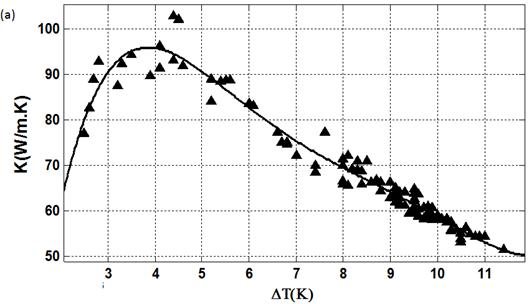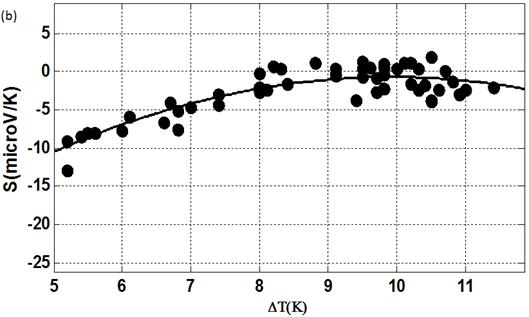Figure 9. Calculated variation for (a) thermal conductivity and (b) Seebeck coefficient vs. temperature difference for the graphite sample.

Apart from the Seebeck coefficient we can also look at the figure of merit (FOM) and the overall efficiency of a material using this model. In this case we have calculated the FOM and the efficiency of the natural graphite sample and the variations with temperature difference are shown in figure 10 (a) and (b).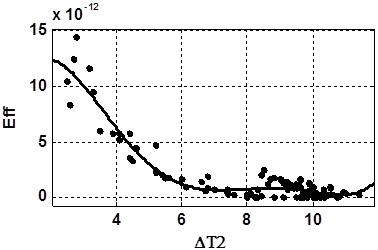Figure 10. Calculated variation for (a) Figure of merit (Z) and (b) Efficiency vs. temperature difference for the graphite sample.

As the two graphs above depict graphite in its raw natural form is not efficient or does not provide adequate figure of merit to use as an energy efficient thermoelectric material. Though this may be used in low tech devices which does not require high efficiency or high output values to operate. As it is cheap and easily found we can also manufacture other forms of graphite such as GICs, nanotubes and graphene all of which have exhibited larger FOM and efficiency vales in previous work.

6. Discussion and Conclusion

The discussed model for calculating the thermoelectric behaviour of a material has been simulated for a laboratory based environment. External errors may exist especially when calculating thermal conductivity of a material using the comparative cut bar method mainly due to thermal insulation issues. The model is also dependent on external data specifically from the MATLAB workspace. Hence, it is also possible to input a reference dataset based on previous work to verify certain output values.

As the resistance values are also obtained thorough a measured dataset we can also extend the model to calculate physical parameters such as the temperature coefficient of resistance. Another advantage of this model is that we can adjust it for a different method for calculating thermal conductivity thus we can use it as a laboratory interface especially for students who are calculating these values. As we are using experimentally obtained datasets the accuracy of the output values is higher and these values can be exported to MATLAB to be further analysed. The model is also user friendly with a dialog box which allows the user to change parameters of the sample as well as include data from a reference material. Future work includes the further verification of this model for more extensive experimental work as well as extension of this model to predict output values when various cooling techniques are used to increase the temperature difference.

Acknowledgements

The authors wish to thank the director and members of the academic and non-academic staff at the National Institute of Fundamental Studies for their gracious support in completing this research work.

References

1. B. Orr, A. Akbarzadeh, M. Mochizuki and R. Singh. "A review of car waste heat recovery systems utilising thermoelectric generators and heat pipes," in Applied Thermal Engineering, 2016, p. 13.
2. F. Felgner, L. Exel, M. Nesarajah, and G. Frey." Component-Oriented Modeling of Thermoelectric Devices for Energy System Design" in IEEE Transactions on Industrial Electronics, vol. 61 (3), 2014, p. 1301-1310
3. Y. Moumouni and R. J. Baker. "Improved SPICE Modeling and Analysis of a Thermoelectric Module," in International Midwest Symposium on Circuits and Systems (MWSCAS), Fort Collins, CO, IEEE, 2015.
4. C. Li, et al, "Thermoelectric Cooling for Power Electronics Circuits: Modeling and Active Temperature Control," IEEE Transactions on Industry Applications, vol. 50 6), 2014, p. 3995 - 4005.
5. A. Kane, V. Verma, and B. Singh. "Temperature Dependent Analysis of Thermoelectric Module using Matlab/SIMULINK," in IEEE International Conference on Power and Energy (PECon), Kota Kinabalu Sabha, Malaysia, 2012.
6. A. M. Yusop, et al., "Dynamic Modeling and Simulation of a Thermoelectric-Solar Hybrid Energy System Using an Inverse Dynamic Analysis Input Shaper" in Modelling and Simulation in Engineering, 2014: p. 13.
7. A. M. Yusop, R. Mohamed and A. Ayob "Model Building of Thermoelectric Generator Exposed to Dynamic Transient Sources" in IOP Conf. Series: Materials Science and Engineering, vol. 53, 2013.
8. B. Ciylan. "Determination of Output Parameters of a Thermoelectric Module using Artificial Neural Networks," in Elektronika ir Elektrotechnika, vol. 116 (10), 2011, p. 63-66.
9. H. L. Tsai and J.-M. Lin, "Model Building and Simulation of Thermoelectric Module Using Matlab/Simulink," Journal Of Electronic Materials, vol. 39 (9), 2010, p. 2105-2111.
10. Y. Apertet and C. Goupil, "On the fundamental aspect of the first Kelvin's relation in thermoelectricity," in International Journal of Thermal Sciences, vol. 104, 2016, p. 225-227.
11. Editors, "Thermal Conductivity - Different Methods for Measurement of Thermal Conductivity," 2011, 11th June 2013 [cited 8th March 2016]; Available from: http://www.azom.com/article.aspx?ArticleID=5615.
12. TA Instruments, "Principal Methods of Thermal Conductivity Measurement," 2012, p. 5.
13. J. Wilson, "Thermal conductivity of solders," in Electronics Cooling, vol. 12 (3), 2006, p. 4-5.
14. S. Desai and J. Njuguna, "Thermal properties of natural graphite flake composites," in International Review of Mechanical Engineering, 4th ed., vol. 6, 2012, p. 923-926.
15. M. Smalc, et al. "Thermal performance of natural graphite heat spreaders," in ASME 2005 Pacific Rim Technical Conference and Exhibition on Integration and Packaging of MEMS, NEMS, and Electronic Systems collocated with the ASME 2005 Heat Transfer Summer Conference, San Francisco, CA, USA, American Society of Mechanical Engineers, 2005.
16. Y. M. Hoi and D. D. L. Chung, "Flexible graphite as a compliant thermoelectric material," in Carbon, vol. 40 (7), 2001, p. 1134-1136.
17. M. Penza, et al., "Thermoelectric Properties of Carbon Nanotubes Layers," in Sensors and Microsystems, vol. 91, 2011, Springer, p. 73-79.
18. R. Matsumoto, Y. Okabe, and N. Akuzawa, "Thermoelectric Properties and Performance of n-Type and p-Type Graphite Intercalation Compounds," in Journal of Electronic Materials, vol. 44 (1), 2015, p. 399-406.
19. R. Javadi, P. H. Choi, H. S. Park, and B. D. Choi, "Preparation and Characterization of P-Type and N-Type Doped Expanded Graphite Polymer Composites for Thermoelectric Applications," Journal of Nanoscience and Nanotechnology, 11th ed., vol. 15, November 2015, p. 9116-9119.

 Contents 1. 2. 3. 4. 5. 6.
Article ToolsAbstractPDF(2808K)